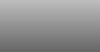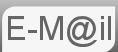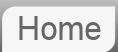# Answer to puzzle #19: 100 Factorial

19. How many consecutive zeros are there at the end of 100! (100 factorial). How would your solution change if the problem were in base 5? How about in Binary???

This is a little tricky, some thinking outside the box needed here...

(The base 5 problem is actually a fair bit easier than the base 10 problem, but for the sake of convention we'll look at the base 10 before the base 5.)

First lets make sure we know what is meant by 100!, 100! = (100 x 99 x 98 x 97 x.... ....x 3 x 2 x 1)

One solution would be to work this out and count the zeros, well I've done this so you don't have to. The answer is...

93,​ 326,​ 215,​ 443,​ 944,​ 152,​ 681,​ 699,​ 238,​ 856,​ 266,​ 700,​ 490,​ 715,​ 968,​ 264,​ 381,​ 621,​ 468,​ 592,​ 963,​ 895,​ 217,​ 599,​ 993,​ 229,​ 915,​ 608,​ 941,​ 463,​ 976,​ 156,​ 518,​ 286,​ 253,​ 697,​ 920,​ 827,​ 223,​ 758,​ 251,​ 185,​ 210,​ 916,​ 864,​ 000,​ 000,​ 000,​ 000,​ 000,​ 000,​ 000,​ 000

Well now you can clearly just count the zeros but actually working out the number is not practical so we need another plan.

The clever bit here is thinking what numbers when multiplied together will end in a zero, if asked you how many zeros were on the end of 3 x 3 x 3 x 3 x 3 x 1,000,000 you wouldn't need to do the sum to know the answer is 6.

So the product of what numbers when multiplied ends in a zero:
1. When one of the things being multiplied ends in zero itself.
2. A number ending in 5 multiplied by an even number.
3. 25, 50 and 75 when multiplied by some of the small numbers available eg (4, 2 and 6) generate an extra zero.
Below is tabulated the origin of all the zeros:

Number Zeros Number Zeros
100 2 95 1
90 1 85 1
80 1 75 2
70 1 65 1
60 1 55 1
50 2 45 1
40 1 35 1
30 1 25 2
20 1 15 1
10 1 5 1
Total 12 Total 12

So that's it then there are 24 zeros on the end of 100!

Another way of thinking of this is with respect to the factors of 5. That is to say the number of times you can divide a number by 5 without getting a non integer result. The table above is in fact an account of all the factors of 5 in the range 1 to 100. Obviously some numbers have more that one factor of 5.

## Now the base 5 problem...

We will employ a technique similar to that we used in base 10, but first lets make sure we know what we mean by base five.

Base five is a counting system where rather than there being 10 available digits (0 - 9) there are 5 (0 - 4) hence the digits of a base 5 number represent from the right, 1's, 5's, 25's, 125's etc. rather than 1's, 10's, 100's, 1000's etc.

So the first few numbers in base 5 are 1, 2, 3, 4, 10, 11, 12, 13, 14, 20, 21... ...43, 44, 100, 101, 102...

Again we have to look at the sources of the zeros, only this time it's much easier. Since 5 is a prime number then there is no way of making a zero other than by multiplying by a number that ends in a zero when written in base 5. You could write down all the numbers and count the zeros. See the table below.

Number
Base 10
Number
Base 5
Zeros Number
Base 10
Number
Base 5
Zeros
100 400 2 95 340 1
90 330 1 85 320 1
80 310 1 75 300 2
70 240 1 65 230 1
60 220 1 55 210 1
50 200 2 45 140 1
40 130 1 35 120 1
30 110 1 25 100 2
20 40 1 15 30 1
10 20 1 5 10 1
Total 12   Total 12

## Now in Binary....

The approach here is going to be roughly the same... We need to think what would cause a zero to be on the end of the product of multiplication.

I propose that like with the base 5 problem, because 2 is prime, (remember binary is base 2,) the only way to make a zero is to multiply by a number that ends in a zero. And therefor the number of zeros on the end of 100! in binary is the sum of the number of zeros on the end of the numbers from 1 to 100 when written in binary.

If this is not clear then think of two binary numbers, multiply them together and see that the numbers of zeros is conserved.

I have designed a spread sheet to convert the numbers 1 to 100 into binary and count up the number of zeros, you will need excel and possibly to have the analytics toolkit installed.

19binary100.xls (49kbytes) - 19binary100.zip (9.7kbytes)

The table, obviously 100 lines of it is below, initially hidden.

So that's it the answer is 97. There is though a more eloquent way of ariving at the sum other than directly counting them. There does seem to be something of a pattern. The trick is to think about the source of the zeros....

A number that has a factor of two will have atleast one zero on the end in the same way as, in base ten a number that has a factor of ten will have at least one zero.

A number that has a factor of four will end in atleast 2 zeros, factor 8 three zeros etc. etc.

The problem is this: because a number is a factor of say 16 and therefor has 4 zeros it we need to be careful not to double count the zeros we will have noted for it being a factor of 8, 4 and 2. Similarly the number may go on to be a factor of 32 and 64 as well.

The Solution to this is that when we note a number is a factor of 2, 4, 8, 16, 32 or 64 we only add one zero knowing that we will already have considered its lower factors and will go on to consider any higher factors.

now we just need to add up the number of factors of 2, 4, 8, 16, 32 & 64

Factors Occurrences
2 50
4 25
8 12
16 6
32 3
64 1
total 97

So there we have it, 97 again! I hate doing tables in HTML.

© Nigel Coldwell 2004 -  – The questions on this site may be reproduced without further permission, I do not claim copyright over them. The answers are mine and may not be reproduced without my expressed prior consent. Please inquire using the link at the top of the page. Secure version of this page.

PayPal
I always think it's arrogant to add a donate button, but it has been requested. If I help you get a job though, you could buy me a pint! - nigel